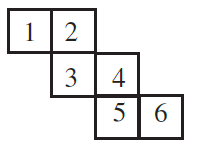# Ex.15.1 Q3 Visualising Solid Shapes - NCERT Maths Class 7

## Question

Can this be a net for a die?Video Solution
Visualizing Solid Shapes
Ex 15.1 | Question 3

## Text Solution

What is known?

A net.

What is unknown?

Can this be a net for a dice.

Reasoning:

Dice is a cube with dots on each face. Opposite faces of a die always have a total of seven dots on them. Make a cube of this net and if opposite faces of this cube have sum of $$7$$ then it can be a net for dice.

Steps:

No, because we know that dice is a cube with dots on each face. Opposite faces of a die always have a total of seven dots on them.

So, if we will fold this net opposite to $$4$$ will be $$1$$ and opposite to $$3$$ will be $$6$$ which does not make total of $$7.$$

Learn from the best math teachers and top your exams

• Live one on one classroom and doubt clearing
• Practice worksheets in and after class for conceptual clarity
• Personalized curriculum to keep up with school